## Fast String Searching With Suffix Trees

### Dr. Dobb's Journal

August, 1996

I think that I shall never see
A poem lovely as a tree.
Poems are made by fools like me,
But only God can make a tree.

--Joyce Kilmer

A tree's a tree. How many more do you need to look at?

--Ronald Reagan-

Matching string sequences is a problem that computer programmers face on a regular basis. Some programming tasks, such as data compression or DNA sequencing, can benefit enormously from improvements in string matching algorithms. This article discusses a relatively unknown data structure, the suffix tree, and shows how its characteristics can be used to attack difficult string matching problems.

The problem

Imagine that you've just been hired as a programmer working on a DNA sequencing project. Researchers are busy slicing and dicing viral genetic material, producing fragmented sequences of nucleotides. They send these sequences to your server, which is then expected to locate the sequences in a database of genomes. The genome for a given virus can have hundreds of thousands of nucleotide bases, and you have hundreds of viruses in your database. You are expected to implement this as a client/server project that gives real-time feedback to the impatient Ph.D.s. What's the best way to go about it?

It is obvious at this point that a brute force string search is going to be terribly inefficient. This type of search would require you to perform a string comparison at every single nucleotide in every genome in your database. Testing a long fragment that has a high hit rate of partial matches would make your client/server system look like an antique batch processing machine. Your challenge is to come up with an efficient string matching solution.

The intuitive solution

Since the database that you are testing against is invariant, preprocessing it to simplify the search seems like a good idea. One preprocessing approach is to build a search trie. For searching through input text, a straightforward approach to a search trie yields a thing called a suffix trie. (The suffix trie is just one step away from my final destination, the suffix tree.) A trie is a type of tree that has N possible branches from each node, where N is the number of characters in the alphabet. The word 'suffix' is used in this case to refer to the fact that the trie contains all of the suffixes of a given block of text (perhaps a viral genome.)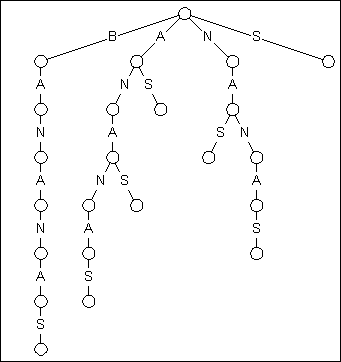Figure 1

The Suffix Trie Representing "BANANAS"

Figure 1 shows a Suffix trie for the word BANANAS. There are two important facts to note about this trie. First, starting at the root node, each of the suffixes of BANANAS is found in the trie, starting with BANANAS, ANANAS, NANAS, and finishing up with a solitary S. Second, because of this organization, you can search for any substring of the word by starting at the root and following matches down the tree until exhausted.

The second point is what makes the suffix trie such a nice construct. If you have a input text of length N, and a search string of length M, a traiditonal brute force search will take as many as N*M character comparison to complete. Optimized searching techniques, such as the Boyer-Moore algorithm can guarantee searches that require no more than M+N comparisons, with even better average performance. But the suffix trie demolishes this performance by requiring just M character comparisons, regardless of the length of the text being searched!

Remarkable as this might seem, it means I could determine if the word BANANAS was in the collected works of William Shakespeare by performing just seven character comparisons! Of course, there is just one little catch: the time needed to construct the trie.

The reason you don't hear much about the use of suffix tries is the simple fact that constructing one requires O(N2) time and space. This quadratic performance rules out the use of suffix tries where they are needed most: to search through long blocks of data.

A reasonable way past this dilemma was proposed by Edward McCreight in 1976, when he published his paper on what came to be known as the suffix tree. The suffix tree for a given block of data retains the same topology as the suffix trie, but it eliminates nodes that have only a single descendant. This process, known as path compression, means that individual edges in the tree now may represent sequences of text instead of single characters.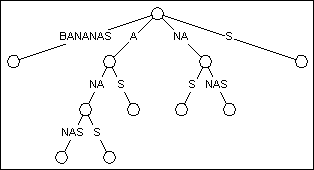Figure 2

A suffix tree representing BANANAS

Figure 2 shows what the suffix trie from Figure 1 looks like when converted to a suffix tree. You can see that the tree still has the same general shape, just far fewer nodes. By eliminating every node with just a single descendant, the count is reduced from 23 to 11.

In fact, the reduction in the number of nodes is such that the time and space requirements for constructing a suffix tree are reduced from O(N2) to O(N). In the worst case, a suffix tree can be built with a maximum of 2N nodes, where N is the length of the input text. So for a one-time investment proportional to the length of the input text, we can create a tree that turbocharges our string searches.

Even you can make a tree

McCreight's original algorithm for constructing a suffix tree had a few disadvantages. Principle among them was the requirement that the tree be built in reverse order, meaning characters were added from the end of the input. This ruled the algorithm out for on line processing, making it much more difficult to use for applications such as data compression.

Twenty years later, Esko Ukkonen from the University of Helsinki came to the rescue with a slightly modified version of the algorithm that works from left to right. Both my sample code and the descriptions that follow are based on Ukkonen's work, published in the September 1995 issue of Algorithmica.

For a given string of text, T, Ukkonen's algorithm starts with an empty tree, then progressively adds each of the N prefixes of T to the suffix tree. For example, when creating the suffix tree for BANANAS, B is inserted into the tree, then BA, then BAN, and so on. When BANANAS is finally inserted, the tree is complete.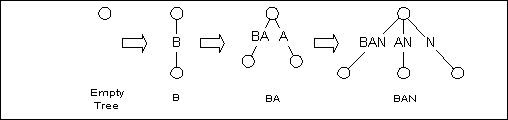Figure 3

Progressively Building the Suffix Tree

Suffix tree mechanics

Adding a new prefix to the tree is done by walking through the tree and visiting each of the suffixes of the current tree. We start at the longest suffix (BAN in Figure 3), and work our way down to the shortest suffix, which is the empty string. Each suffix ends at a node that consists of one of these three types:

1. A leaf node. In Figure 4, the nodes labeled 1,2, 4, and 5 are leaf nodes.
2. An explicit node. The non-leaf nodes that are labeled 0 and 3 in Figure 4 are explicit nodes. They represent a point on the tree where two or more edges part ways.
3. An implicit node. In Figure 4, prefixes such as BO, BOO, and OO all end in the middle of an edge. These positions are referred to as implicit nodes. They would represent nodes in the suffix trie, but path compression eliminated them. As the tree is built, implicit nodes are sometimes converted to explicit nodes.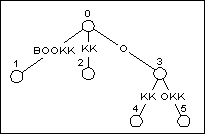Figure 4

In Figure 4, there are five suffixes in the tree (including the empty string) after adding BOOK to the structure. Adding the next prefix, BOOKK to the tree means visiting each of the suffixes in the existing tree, and adding letter K to the end of the suffix.

The first four suffixes, BOOK, OOK, OK, and K, all end at leaf nodes. Because of the path compression applied to suffix trees, adding a new character to a leaf node will always just add to the string on that node. It will never create a new node, regardless of the letter being added.

After all of the leaf nodes have been updated, we still need to add character 'K' to the empty string, which is found at node 0. Since there is already an edge leaving node 0 that starts with letter K, we don't have to do anything. The newly added suffix K will be found at node 0, and will end at the implicit node found one character down along the edge leading to node 2.

The final shape of the resulting tree is shown in Figure 5.Figure 5

The same tree after adding BOOKK

Things get knotty

Updating the tree in Figure 4 was relatively easy. We performed two types of updates: the first was simply the extension of an edge, and the second was an implicit update, which involved no work at all. Adding BOOKKE to the tree shown in Figure 5 will demonstrate the two other types of updates. In the first type, a new node is created to split an existing edge at an implicit node, followed by the addition of a new edge. The second type of update consists of adding a new edge to an explicit node.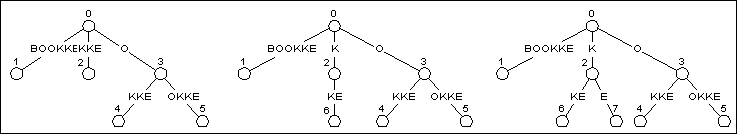Figure 6

When adding BOOKKE to the tree in Figure 5, we once again start with the longest suffix, BOOKK, and work our way to the shortest, the empty string. Updating the longer suffixes is trivial as long as we are updating leaf nodes. In Figure 5, the suffixes that end in leaf nodes are BOOKK, OOKK, OKK, and KK. The first tree in Figure 6 shows what the tree looks like after these suffixes have been updated using the simple string extension.

The first suffix in Figure 5 that doesn't terminate at a leaf node is K. When updating a suffix tree, the first non-leaf node is defined as the active point of the tree. All of the suffixes that are longer than the suffix defined by the active point will end in leaf nodes. None of the suffixes after this point will terminate in leaf nodes.

The suffix K terminates in an implicit node part way down the edge defined by KKE. When testing non-leaf nodes, we need to see if they have any descendants that match the new character being appended. In this case, that would be E.

A quick look at the first K in KKE shows that it only has a single descendant: K. So this means we have to add a descendent to represent Letter E. This is a two step process. First, we split the edge holding the arc so that it has an explicit node at the end of the suffix being tested. The middle tree in Figure 6 shows what the tree looks like after the split.

Once the edge has been split, and the new node has been added, you have a tree that looks like that in the third position of Figure 6. Note that the K node, which has now grown to be KE, has become a leaf node.

Updating an explicit node

After updating suffix K, we still have to update the next shorter suffix, which is the empty string. The empty string ends at explicit node 0, so we just have to check to see if it has a descendant that starts with letter E. A quick look at the tree in Figure 6 shows that node 0 doesn't have a descendent, so another leaf node is added, which yields the tree shown in Figure 7.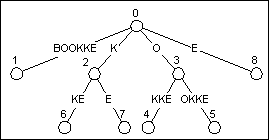Figure 7

Generalizing the algorithm

By taking advantage of a few of the characteristics of the suffix tree, we can generate a fairly efficient algorithm. The first important trait is this: once a leaf node, always a leaf node. Any node that we create as a leaf will never be given a descendant, it will only be extended through character concatenation. More importantly, every time we add a new suffix to the tree, we are going to automatically extend the edges leading into every leaf node by a single character. That character will be the last character in the new suffix.

This makes management of the edges leading into leaf nodes easy. Any time we create a new leaf node, we automatically set its edge to represent all the characters from its starting point to the end of the input text. Even if we don't know what those characters are, we know they will be added to the tree eventually. Because of this, once a leaf node is created, we can just forget about it! If the edge is split, its starting point may change, but it will still extend all the way to the end of the input text.

This means that we only have to worry about updating explicit and implicit nodes at the active point, which was the first non-leaf node. Given this, we would have to progress from the active point to the empty string, testing each node for update eligibility.

However, we can save some time by stopping our update earlier. As we walk through the suffixes, we will add a new edge to each node that doesn't have a descendant edge starting with the correct character. When we finally do reach a node that has the correct character as a descendant, we can simply stop updating. Knowing how the construction algorithm works, you can see that if you find a certain character as a descendent of a particular suffix, you are bound to also find it as a descendent of every smaller suffix.

The point where you find the first matching descendent is called the end point. The end point has an additional feature that makes it particularly useful. Since we were adding leaves to every suffix between the active point and the end point, we now know that every suffix longer than the end point is a leaf node. This means the end point will turn into the active point on the next pass over the tree!

By confining our updates to the suffixes between the active point and the end point, we cut way back on the processing required to update the tree. And by keeping track of the end point, we automatically know what the active point will be on the next pass. A first pass at the update algorithm using this information might look something like this (in C-like pseudo code) :

```Update( new_suffix )
{
current_suffix = active_point
test_char = last_char in new_suffix
done = false;
while ( !done ) {
if current_suffix ends at an explicit node {
if the node has no descendant edge starting with test_char
create new leaf edge starting at the explicit node
else
done = true;
} else {
if the implicit node's next char isn't test_char {
split the edge at the implicit node
create new leaf edge starting at the split in the edge
} else
done = true;
}
if current_suffix is the empty string
done = true;
else
current_suffix = next_smaller_suffix( current_suffix )
}
active_point = current_suffix
}

```

The Suffix Pointer

The pseudo-code algorithm shown above is more or less accurate, but it glosses over one difficulty. As we are navigating through the tree, we move to the next smaller suffix via a call to next_smaller_suffix(). This routine has to find the implicit or explicit node corresponding to a particular suffix.

If we do this by simply walking down the tree until we find the correct node, our algorithm isn't going to run in linear time. To get around this, we have to add one additional pointer to the tree: the suffix pointer. The suffix pointer is a pointer found at each internal node. Each internal node represents a sequence of characters that start at the root. The suffix pointer points to the node that is the first suffix of that string. So if a particular string contains characters 0 through N of the input text, the suffix pointer for that string will point to the node that is the termination point for the string starting at the root that represents characters 1 through N of the input text.

Figure 8 shows the suffix tree for the string ABABABC. The first suffix pointer is found at the node that represents ABAB. The first suffix of that string would be BAB, and that is where the suffix pointer at ABAB points. Likewise, BAB has its own suffix pointer, which points to the node for AB.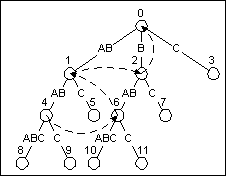Figure 8

The suffix tree for ABABABC with suffix pointers shown as dashed lines

The suffix pointers are built at the same time the update to the tree is taking place. As I move from the active point to the end point, I keep track of the parent node of each of the new leaves I create. Each time I create a new edge, I also create a suffix pointer from the parent node of the last leaf edge I created to the current parent edge. (Obviously, I can't do this for the first edge created in the update, but I do for all the remaining edges.)

With the suffix pointers in place, navigating from one suffix to the next is simply a matter of following a pointer. This critical addition to the algorithm is what reduces it to an O(N) algorithm.

Tree houses

To help illustrate this article, I wrote a short program, STREE.CPP, that reads in a string of text from standard input and builds a suffix tree. The version shown in Listing 1, is fully documented C++. A second version, STREED.CPP, has extensive debug output as well, and is available from the DDJ listing service, as well as my home page.

Understanding STREE.CPP is really just a matter of understanding the workings of the data structures that it contains. The most important data structure is the Edge object. The class definition for Edge is:

```class Edge {
public :
int first_char_index;
int last_char_index;
int end_node;
int start_node;
void Insert();
void Remove();
Edge();
Edge( int init_first_char_index,
int init_last_char_index,
int parent_node );
int SplitEdge( Suffix &s );
static Edge Find( int node, int c );
static int Hash( int node, int c );
};
```

Each time a new edge in the suffix tree is created, a new Edge object is created to represent it. The four data members of the object are defined as follows:

 first_char_index, last_char_index: Each of the edges in the tree has a sequence of characters from the input text associated with it. To ensure that the storage size of each edge is identical, we just store two indices into the input text to represent the sequence. start_node: The number of the node that represents the starting node for this edge. Node 0 is the root of the tree. end_node: The number of the node that represents the end node for this edge. Each time an edge is created, a new end node is created as well. The end node for every edge will not change over the life of the tree, so this can be used as an edge id as well.

One of the most frequent tasks performed when building the suffix tree is to search for the edge emanating from a particular node based on the first character in its sequence. On a byte oriented computer, there could be as many as 256 edges originating at a single node. To make the search reasonably quick and easy, I store the edges in a hash table, using a hash key based on their starting node number and the first character of their substring. The Insert() and Remove() member functions are used to manage the transfer of edges in and out of the hash table.

The second important data structure used when building the suffix tree is the Suffix object. Remember that updating the tree is done by working through all of the suffixes of the string currently stored in the tree, starting with the longest, and ending at the end point. A Suffix is simply a sequence of characters that starts at node 0 and ends at some point in the tree.

It makes sense that we can then safely represent any suffix by defining just the position in the tree of its last character, since we know the first character starts at node 0, the root. The Suffix object, whose definition is shown here, defines a given suffix using that system:

```    class Suffix {
public :
int origin_node;
int first_char_index;
int last_char_index;
Suffix( int node, int start, int stop );
int Explicit();
int Implicit();
void Canonize();
};
```

The Suffix object defines the last character in a string by starting at a specific node, then following the string of characters in the input sequence pointed to by the first_char_index and last_char_index members. For example, in Figure 8, the longest suffix "ABABABC" would have an origin_node of 0, a first_char_index of 0, and a last_char_index of 6.

Ukkonen's algorithm requires that we work with these Suffix definitions in canonical form. The Canonize() function is called to perform this transformation any time a Suffix object is modified. The canonical representation of the suffix simply requires that the origin_node in the Suffix object be the closest parent to the end point of the string. This means that the suffix string represented by the pair (0, "ABABABC"), would be canonized by moving first to (1, "ABABC"), then (4, "ABC"), and finally (8,"").

When a suffix string ends on an explicit node, the canonical representation will use an empty string to define the remaining characters in the string. An empty string is defined by setting first_char_index to be greater than last_char_index. When this is the case, we know that the suffix ends on an explicit node. If first_char_index is less than or equal to last_char_index, it means that the suffix string ends on an implicit node.

Given these data structure definitions, I think you will find the code in STREE.CPP to be a straightforward implementation of the Ukkonen algorithm. For additional clarity, use STREED.CPP to dump copious debug information out at runtime.

Acknowledgments

I was finally convinced to tackle suffix tree construction by reading Jesper Larsson's paper for the 1996 IEEE Data Compression Conference. Jesper was also kind enough to provide me with sample code and pointers to Ukkonen's paper.

References

E.M. McCreight. A space-economical suffix tree construction algorithm. Journal of the ACM, 23:262-272, 1976.

E. Ukkonen. On-line construction of suffix trees. Algorithmica, 14(3):249-260, September 1995.

Source Code

 stree.cpp A simple program that builds a suffix tree from an input string. streed.cpp The same program with much debugging code added.